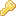The `%CALC{"formula"}%` macro is handled by the SpreadSheetPlugin. There are around 90 formulae, such as `\$ABS()`, `\$EXACT()`, `\$EXISTS()`, `\$GET()/\$SET()`, `\$IF()`, `\$LOG()`, `\$LOWER()`, `\$PERCENTILE()`, `\$TIME()`, `\$VALUE()`.
• This macro is specifically for manipulating data in tables, and so is evaluated after the normal Macro expansion order. If you need a standard ordered evaluation see CALCULATE

## Examples

• `%CALC{"\$SUM(\$ABOVE())"}%` returns the sum of all cells above the current cell
• `%CALC{"\$EXISTS(Web.SomeTopic)"}%` returns `1` if the topic exists
• `%CALC{"\$UPPER(Collaboration)"}%` returns `COLLABORATION`

•Log In